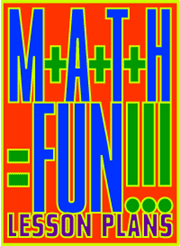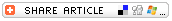## Search formReturn to Math Lessons
Math MagicSubjects

• Mathematics
--Arithmetic

• 3-5
• 6-8
• 9-12

Brief Description

Students figure out people's ages by doing simple math calculations.

Objectives

Students will

• reinforce math computation skills by doing fun activities with their peers or adult acquaintances.

Keywords

age, arithmetic, birthday, calculate, math, puzzle, year

• pencil and paper

Lesson Plan

This lesson includes two puzzle activities students can do to exercise their math skills and impress their parents, neighbors, and relatives. In both activities, kids provide adults with directions that require simple math computations in order to help the kids -- magically! -- figure out the ages of the other people. Following are the directions students will provide as they work these two puzzles on their peers or adult acquaintances.

Puzzle 1 (for students who can do simple addition and subtraction and multiply by 5)

• Ask an adult whether he or she would be willing to do a math puzzle that will enable you to figure out how old he or she is without even asking his or her age!
• If the adult agrees, ask him or her to think of the first number in his or her age. (For example, if the person is 36 years old, she or he will think the number 3.)
• Tell the person to multiply that number by 5.
• Then tell the person to add 3 to that number.
• Finally, ask the adult to add the second number of his or her age to the total. For example, if the person is 36 years old, he or she will add 6 to the number in the previous step.
• Ask the adult to tell you the number that resulted from the calculations.
• Now, simply subtract 6 from that number. Announce the answer as that person's age!

Encourage students to try this puzzle in advance a few times -- using random numbers (ages) -- to see whether it works. That will provide math practice for students. For this activity, you might provide, or students might create, a simple chart with the following headings:

• Person's Age
• First Number of the Person's Age
• Multiply x 5
• Double the Number
• Add the Second Number of the Person's Age
• Subtract 6 (To Get the Person's Age)

Challenge Question: See whether students can figure out why the puzzle works!

Puzzle 2 (for students who can add larger numbers and multiply by 50)

• Ask an adult whether she or he would be willing to do a math puzzle that will enable you to figure out how old he or she is without even asking her or his age!
• If the adult agrees, ask him or her to think of a number -- any number.
• Ask the person to double that number.
• Then tell the person to add 5 to the number.
• Now tell the adult to multiply the number by 50.
• Ask the adult whether she or he has had a birthday so far this year. If so, tell the adult to add 1752* to the number. If the person has not celebrated a birthday yet this year, tell the person to add 1751.*
• Finally, ask the person to subtract the year (for example, 1952 or 1979) of birth.
• The number the adult has now should tell you two things: The first digit or digits will be the original number that the adult started with; the last two digits will be the person's age!
Encourage students to try this puzzle on their peers to verify that it works. Doing this will provide math practice.

Challenge Question: See if students can figure out why the puzzle works!

Note: The numbers 1752 and 1751 will work only in the year 2002. If you are doing this puzzle activity in 2003, add 1 to each of those numbers (use 1753 and 1752); if you are doing the puzzle in 2004, add 2 to each of those numbers (use 1754 and 1753) and so on.

Assessment

Students do a series of calculations to help them see that these puzzles work. If they come across an example that does not work, they redo the calculation to find their error(s).

Lesson Plan Source

Education World

Submitted By

Gary Hopkins

National Standards

MATHEMATICS: Number and Operations
NM-NUM.3-5.3 Compute Fluently and Make Reasonable Estimates
NM-NUM.6-8.3 Compute Fluently and Make Reasonable Estimates